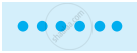# Arranging the objects in rows and columns:

Ram has 6 marbles with him. He wants to arrange them in rows in such a way that each row has the same number of marbles. He arranges them in the following ways and matches the total number of marbles.

### Arrangement

1) 1 marble in each row
Number of rows = 6
Total number of marbles = 1 × 6 = 6.2) 3 marbles in each row
Number of rows = 2
Total number of marbles = 3 × 2 = 6.3) 6 marblesin a row.
Number of rows = 1
Total number of marbles = 6 × 1 = 6.4) 2 marbles in each row
Number of rows = 3
Total number of marbles = 2 × 3 = 6From these calculations Ramesh observes that 6 can be written as a productof two numbers in different ways as 6 = 1 × 6; 6 = 2 × 3; 6 = 3 × 2; 6 = 6 × 1

From 6 = 2 × 3 it can be said that 2 and 3 exactly divide 6. So, 2 and 3 are the exact divisors of 6. From the other product 6 = 1 × 6, the exact divisors of 6 are found to be 1 and 6. Thus, 1, 2, 3, and 6 are exact divisors of 6. They are called the factors of 6.

If you would like to contribute notes or other learning material, please submit them using the button below.